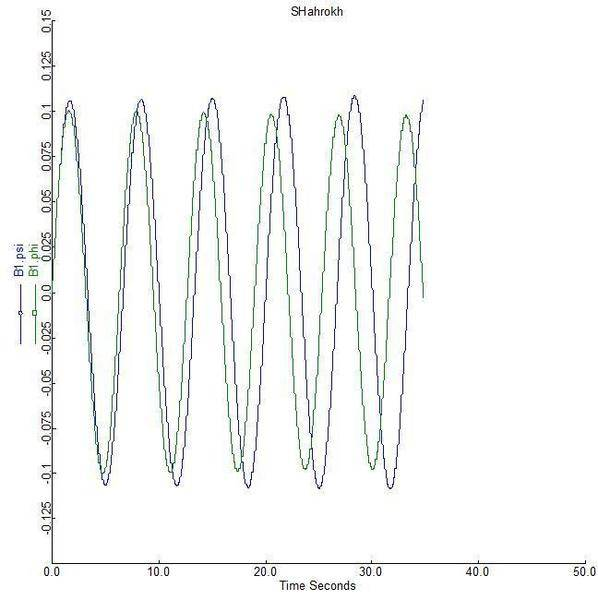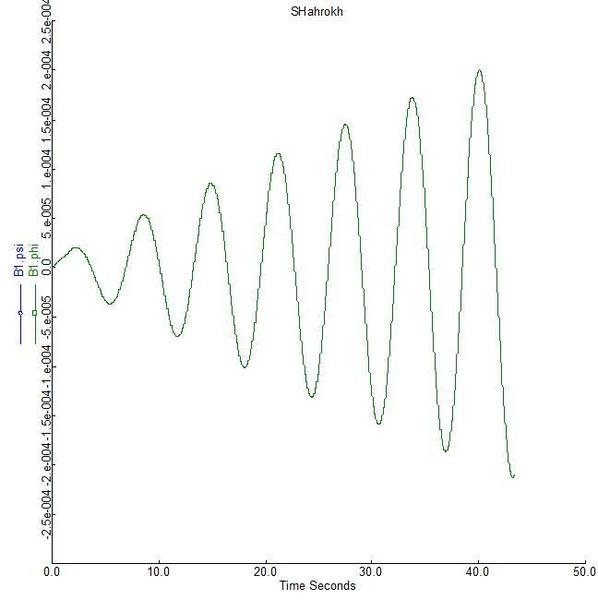# Coupled differential equation with boundary conditions

Hi,
I have two coupled differential equations
d^2 phi(z)/dz^2=lambda*phi(z)*(phi(z)^2+psi(z)^2-sigma^2)
d^2 psi(z)/dz^2=lambda*psi(z)*(phi(z)^2+psi(z)^2-sigma^2+epsilon/lambda)
where lambda, epsilon and sigma are arbitrary constants. The equation subject to the bellow boundary conditions
phi(0)=0, phi(infinity)=sigma, psi(infinity)=0,
I couldn't find any analytical solution but how can I solve them numerically, if I use infinity as the initial point then the answer is the trivial phi(z)=sigma and psi(z)=0 which doesn't satisfy the third boundary condition but I know that this equation must has a solution since a non differential solution is already at my disposal . Thanks.

BvU
Homework Helper
Hello Shahrokh, welcome to PF!

Let me rephrase:$${\partial^2 \phi \over \partial z^2} = \lambda \phi \left ( \phi^2 + \psi^2 - \sigma^2 \right ) \\ \mathstrut\\ {\partial^2 \psi \over \partial z^2} = \lambda \psi \left ( \phi^2 + \psi^2 - \sigma^2+ {\epsilon\over \lambda} \right ) \\ \mathstrut\\ \quad \quad \phi(0) = 0, \quad \phi(\infty)=\sigma, \quad \psi(\infty)=0$$
and then I miss a fourth (initial) condition, but in the text you mention using ##\infty## as initial point (how do you do that ?) that would lead to ##\phi = \sigma## and ##\psi = 0## . I take it you set ##\psi(0)## to a large number ?

I suppose ##\epsilon## is a small number ? And ##\lambda, \ \sigma## can be set to 1 for a demo ? Or do you have values ?

Just for clarity: with ##\phi(0) = 0##, ##{d\phi\over dz}(0) \ne 0## you really need a very small value for this derivative to keep it from running away ?

--

If I just let my integration tool run with invented values

Code:
eps  as realparameter (0.1)  ;
lambda  as realparameter (1)  ;
s_sigma  as realparameter (1)  ;

phi  as realvariable (initial, 0)  ;
psi  as realvariable (initial, 0)  ;
dphi_dz  as realvariable (initial, eps)  ;
dpsi_dz  as realvariable (initial, eps)  ;

$phi = dphi_dz ;$psi = dpsi_dz  ;

$dphi_dz = lambda * phi * (phi^2 + psi^2 - s_sigma^2) ;$dpsi_dz = lambda * psi * (phi^2 + psi^2 - s_sigma^2 + eps/lambda)  ;

I getfor eps = 0.1 andfor eps = 1e-5

It will be quite a job to use a shooting method (*) and get the boundary condition fulfilled...

(*) Only method I have in my toolLast edited:
phyzguy
You need to expand phi and psi for large values of z, to get a functional form for how phi and psi deviate from their values at infinity as z gets smaller. Then you can use the shooting method. Write:
$$\varphi \approx \sigma - \alpha f_1(z)$$
$$\psi \approx \alpha f_2(z)$$
If you then plug these in to the differential equations and keep terms to first order in alpha, you will find that both f1 and f2 have the form K exp(- beta z) for some value of beta and some content K. You can then use numerical methods, starting at large values of z and integrating back toward zero, varying the constants K until you meet the boundary conditions at zero. As BvU said, it seems you are mission one more boundary condition. With what you have given, I think you will get a whole family of solutions.

To be more specific this is a domain wall solution and I have already had the answer using minimum total energy. I have also solved it analytically invoking some approximations. If the wall location be at z=0 the above equations are the minkowskian static Klien-Gordon equations. and probably now you get from where the boundary conditions appear: After the fields settle down in one of two discrete vacua we will encounter a static solution, what I am trying to find. Far from the wall the fields behavior is known phi(+infty)=sigma, phi(-infty)=-sigma so \phi(z=0)=0, \psi(\pm infty)=0, but behavior of transition between vacua is the matter of interest. My approximated solution predicts tanh for phi and sech for psi which are somehow convincing but to approve needs a numerical confirmation. There is no reason for epsilon to be small at all and phi and psi are mostly more than one but less than \sigma if one consider the inflation paradigm to be compatible with the proposal. As much as I know one can map infinity into some attainable range for numerical solutions. If one reduce the above equations to their first integrals then only two initial condition suffice to run a numerical procedure which I choose the initial point to be my virtual infinity say z=100. then the solutions are psi=0, phi=sigma which don't satisfy the remain boundary condition at the z=0.
Thanks again.

Chestermiller
Mentor
If I were working on this problem, I would make a change in variables as follows:

##φ=Pσ##

##ψ=Sσ##

##z=\frac{Z}{σ\sqrt{λ}}##

What do you get when you make these substitutions into the equations?

Chet

phyzguy
If you do what ChesterMiller suggested, you will eliminate all of the constants except one, giving:
$$P'' = P(P^2 + S^2 -1); S'' = S(P^2 + S^2 - 1 +\alpha); \alpha = \frac{\epsilon}{\sigma^2 \lambda}$$

Then if you do what I suggested and find solutions for P and S at large z, you will find:
$$P \approx 1 - A e^{-\sqrt{2} z} ; S \approx B e^{-\sqrt{\alpha} z}$$

Now you can start at some large value of z (I used z = 10.0) and integrate backwards toward zero. You then search for values of A and B that meet your boundary condition P(0) = 0. This is called "shooting". Of course, these depend on the value of alpha - I took alpha = 0.5 just to start somewhere. But the problem is still undetermined - there are many values of A and B that will meet your third boundary condition. You need one more boundary condition - either S(0), S'(0), or P'(0).

I uploaded a plot of a numerical solution. This is for alpha = 0.5, A = 2.0, B = 0.1 and it is very close to giving P(0) = 0. It gives S(0) = 0.05.

#### Attachments

Last edited:
Well, thank you your graph shows very good similarity to tanh and sech which are my analytical solutions, except for their amplitude which has to be the matter of choosing the parameters and the missing boundary value, anyway at this stage it was a great help. I will pursue your comments. Thank you a lot.

Last edited:
phyzguy